# tkinter的Canvas组件，讲解演示动画知识初步

Canvas组件，我们可以画线，画圆，绘图等，怎样让这些线，圆，图这些画布的组件动起来呢，目前有3个方法：coords() , itemconfig() , move() ，下面，我就来讲解一下这3种方法。

move ( 对象ID , X方面移动距离,  Y方向移动距离)

```from tkinter import *
import time

root=Tk()

can1 = Canvas(root,width=300, height=200,background='white') # 白底画布
can1.pack(fill=BOTH,expand=True)

can1.create_line(60,20,200,20) # 画线
can1.create_oval(60,50,80,70,outline='red',fill='blue') # 画圆
img1=PhotoImage(file='16-1.png')
can1.create_image(60,120,image=img1) # 绘图

for i in range(50):    # 移动50步
can1.move(1,2,3)   # ID为1,每步:X方向右移动2像素,Y方向不移动
can1.move(2,2,3)  # ID为2,每步:X方向左移动2像素,Y方向不移动
can1.move(3,2,3)   # ID为3,每步:X方向移动2像素,Y像素移动3像素
# can1.move(1,2,0)    # ID为1,线，向左移动
# can1.move(2,-2,0)   # ID为2,圆，向右移动
# can1.move(3,-2,-3)  # ID为3,图，向右上角移动
can1.update()     # 刷新画布
time.sleep(0.05)  # 时间暂停

root.mainloop()```

上面的代码，在画布上创建3个对象：线，圆，图片（它们的ID号，系统会根据创建时间的先后，分配的ID号分别是123），然后通过循环50次，让3个对象，在每一次循环时，X方面向左移动2个像素，Y方向向下移动3个像素。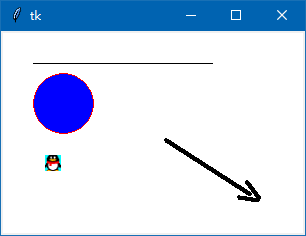can1.update() # 不加这条代码，画布不刷新，我们看不到对象的移动

time.sleep(0.05) # 不暂停一下，我们的眼睛看不到移动过程，由于移动速度太快，只要看到对象最终的移动位置。

move ( 对象ID , X方面移动距离,  Y方向移动距离)

X方面移动距离：为正值是向右移动，为负值是向左移动，为0不移动

Y方面移动距离：为正值是向下移动，为负值是向上移动，为0不移动

```can1.move(1,2,0)    # ID为1,线，向左移动
can1.move(2,-2,0)   # ID为2,圆，向右移动
can1.move(3,-2,-3)  # ID为3,图，向右上角移动```

```from tkinter import *
import time

root=Tk()

can1 = Canvas(root,width=300, height=200,background='white') # 白底画布
can1.pack(fill=BOTH,expand=True)

AAA=can1.create_line(60,20,200,20) # 画线
BBB=can1.create_oval(60,50,80,70,outline='red',fill='blue') # 画圆
img1=PhotoImage(file='16-1.png')
CCC=can1.create_image(60,120,image=img1) # 绘图

for i in range(50):    # 移动50步
can1.move(AAA,2,3)   # ID为1,每步:X方向右移动2像素,Y方向不移动
can1.move(BBB,2,3)  # ID为2,每步:X方向左移动2像素,Y方向不移动
can1.move(CCC,2,3)   # ID为3,每步:X方向移动2像素,Y像素移动3像素
can1.update()     # 刷新画布
time.sleep(0.05)  # 时间暂停

root.mainloop()```

```print(can1.coords(AAA)) # 返回线的坐标
print(can1.coords(BBB)) # 返回圆的坐标
print(can1.coords(CCC)) # 返回图的坐标```

[160.0, 170.0, 300.0, 170.0]

[160.0, 200.0, 180.0, 220.0]

[160.0, 270.0]

```from tkinter import *
import time

root=Tk()

can1 = Canvas(root,width=300, height=200,background='white') # 白底画布
can1.pack(fill=BOTH,expand=True)

AAA=can1.create_line(60,20,200,20) # 画线
BBB=can1.create_oval(60,50,80,70,outline='red',fill='blue') # 画圆
img1=PhotoImage(file='16-1.png')
CCC=can1.create_image(60,120,image=img1) # 绘图

for i in range(50):    # 移动50步
wz1=can1.coords(AAA) # 获取线条目前的位置(x1,y1,x2,y2)
can1.coords(AAA,wz1+3,wz1+3,wz1+3,wz1+3)
# 在线条坐标的基础上,分别+3个像素,让线条向右下角移动

wz2=can1.coords(BBB) # 获取小球目前的位置(x1,y1,x2,y2)
can1.coords(BBB,wz2+3,wz2+3,wz2+3,wz2+3)
# 在小球坐标的基础上,分别+3个像素,让小球向右下角移动

wz3=can1.coords(CCC) # 获取图片目前的位置(x1,y1)
can1.coords(CCC,wz3+3,wz3+3)
# 在图片坐标的基础上,分别+3个像素,让图片向右下角移动

can1.update()     # 刷新画布
time.sleep(0.05)  # 时间暂停

root.mainloop()```1.  对象ID：如系统自动分配的ID123 还有我们自定义的IDAAA,BBB,CCC

2.  tags 为画布对象起的标签名。（下面我会讲解）

3.  ‘all’ :   表示Canvas组件中的所有画布对象，系统内置标签

4.  ‘current’ :  表示鼠标指针下的画布对象，，系统内置标签

`can1.tag_bind(AAA,"<B1-Motion>",test1)`

```
from tkinter import *

root=Tk()

can1 = Canvas(root,width=300, height=200,background='white') # 白底画布
can1.pack(fill=BOTH,expand=True)

AAA=can1.create_line(60,20,200,20,tags='t1') # 画线 加入tags参数
BBB=can1.create_oval(60,50,80,70,outline='red',fill='blue',tags='t2') # 画圆
img1=PhotoImage(file='16-1.png')
CCC=can1.create_image(60,120,image=img1,tags='t3') # 绘图

def test1(event):
can1.coords(AAA,event.x-70,event.y,event.x+70,event.y)  # 用AAA指定对象
#  can1.coords(1,event.x-70,event.y,event.x+70,event.y)    # 用1指定对象也可以
#  can1.coords('t1',event.x-70,event.y,event.x+70,event.y) # 用't1' 指定对象也可以
def test2(event):
can1.coords(BBB,event.x-10,event.y-10,event.x+10,event.y+10) # 用coords方法让对象跟鼠标坐标一同变化
def test3(event):
can1.coords(CCC,event.x,event.y) # 图片坐标只有2个参数，上面的线，小圆有4个参数

can1.tag_bind(AAA,"<B1-Motion>",test1) # 线条 鼠标按下移动事件 绑定函数test1
can1.tag_bind(BBB,"<B1-Motion>",test2) # 小圆 鼠标按下移动事件 绑定函数test2
can1.tag_bind(CCC,"<B1-Motion>",test3) # 图片 鼠标按下移动事件 绑定函数test3

root.mainloop()```

上面代码，3个画布对象分别绑定“按住鼠标左键移动”事件，在绑定的函数里，用coords( 画布对象ID，对象要移动的目的坐标 ) 来移动画布对象。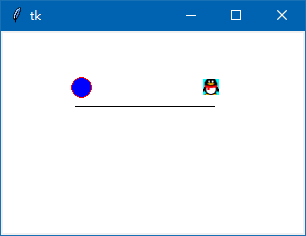```from tkinter import *
import time

def ok(): # 点击按钮，由yn变量决定是暂停还是继续动起来
global yn
if yn == True:
yn = False
but1['text']='  动起来  '
else:
yn = True
but1['text']='  暂 停  '
ballmove()  # 小球继续动起来

def ballmove(): # 小球在画布四周不停反弹
global x, y
while yn: # 由yn变量来控制小球是否移动
can1.move(xq, x, y)         # 初始化,小球向右下角移动
weizhi = can1.coords(xq)    # 获取小球的位置，一个4个元素的元组

if weizhi <= 0:       # 侦测球是否超过画布左方
x = step
if weizhi <= 0:       # 侦测球是否超过画布上方
y = step
if weizhi >= can1width:     # 侦测球是否超过画布右方
x = -step
if weizhi >= can1height:    # 侦测球是否超过画布下方
y = -step

can1.update()       # 刷新画布
time.sleep(speed)   # 可以控制移动速度

root = Tk()

can1width = 200       # 定义画布宽度
can1height = 150      # 定义画布高度
step = 3        # 小于移动的步长,3个像素
x,y=3,3         # 小球移动的初始步长
speed = 0.03    # 设置移动速度
yn = False      # yn的值来控制球是否移动

can1 = Canvas(root, background='lightblue', width=can1width, height=can1height) # 创建画布
can1.pack()

but1 = Button(root, text="  动起来  ", command=ok) # 创建按钮
but1.pack()

xq = can1.create_oval(20, 20, 40, 40, fill='red', outline='green') # 绘制小球

def xq_move(event): # 鼠标拖动小球
can1.coords(xq,event.x-10,event.y-10,event.x+10,event.y+10)
# 将鼠标当前位置转为小球的外接正方形的左上角和右下角坐标（小球的半径为10）

can1.tag_bind(xq,"<B1-Motion>",xq_move) # 小球,鼠标按住移动事件

root.mainloop()```

在上面的代码中，weizhi = can1.coords(xq) 会得到一个元组（x1,y1,x2,y2），x1,y1这是小球外接正方形的左上角坐标，x2,y2是右下角坐标。

x1，即weizi，用于判断是否撞到画布的左边

y1  weizi，用于判断是否撞到画布的上边

x2  weizi，用于判断是否撞到画布的右边

y2  weizi，用于判断是否撞到画布的下边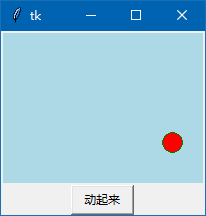```from tkinter import *
import time

def ok(): # 点击按钮，由yn变量决定是暂停还是继续动起来
global yn
if yn == True:
yn = False
but1['text']='  动起来  '
else:
yn = True
but1['text']='  暂 停  '
ballmove()  # 小球继续动起来

def ballmove(): # 小球在画布四周不停反弹
global x, y
while yn: # 由yn变量业控制小球是否移动
can1.move(xq, x, y)        # 初始化,小球向右下角移动
weizhi = can1.coords(xq)   # 获取小球的位置，注意，是2个元素的元组

if weizhi -8 <= 0:          # 侦测球是否超过画布左方
x = step
if weizhi -8 <= 0:          # 侦测球是否超过画布上方
y = step
if weizhi + 8 >= can1width:  # 侦测球是否超过画布右方
x = -step
if weizhi + 8 >= can1height: # 侦测球是否超过画布下方
y = -step

can1.update()       # 刷新画布
time.sleep(speed)   # 可以控制移动速度

root = Tk()

can1width = 200       # 定义画布宽度
can1height = 150      # 定义画布高度
step = 3        # 小于移动的步长,3个像素
x,y=3,3         # 小球移动的初始步长
speed = 0.03    # 设置移动速度
yn = False      # yn的值来控制球是否移动

can1 = Canvas(root, background='lightblue', width=can1width, height=can1height) # 创建画布
can1.pack()

but1 = Button(root, text="  动起来  ", command=ok) # 创建按钮
but1.pack()

img1=PhotoImage(file='16-1.png')
xq=can1.create_image(20,20,image=img1) # 绘制小球图片（现暂用QQ图片代替）

def xq_move(event): # 鼠标拖动小球图片（现暂用QQ图片代替）
can1.coords(xq,event.x,event.y)

can1.tag_bind(xq,"<B1-Motion>",xq_move) # 小球（QQ图片）,鼠标按住移动事件

root.mainloop()```

x1 = weizhi - 8      y1 = weizhi - 8

x2 = weizhi + 8      y2 = weizhi + 8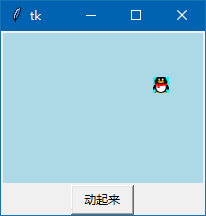```from tkinter import *
import time

def ok():  # 点击按钮，由yn变量决定是暂停还是继续动起来
global yn
if yn == True:
yn = False
but1['text'] = '  动起来  '
else:
yn = True
but1['text'] = '  暂 停  '
ballmove()  # 小球继续动起来

def ballmove():  # 小球在画布四周不停反弹
global x1, y1, x2, y2, yn

while yn:  # 由yn变量来控制小球是否移动

can1.move(xq1, x1, y1)         # 初始化,小球1,红球，向右下角移动
can1.move(xq2, x2, y2)         # 初始化,小球2，蓝球，向右上角移动

wz1 = can1.coords(xq1)   # 获取小球1的位置，一个4个元素的元组

if wz1 <= 0:          # 侦测球1是否超过画布左方
x1 = step
if wz1 <= 0:          # 侦测球1是否超过画布上方
y1 = step
if wz1 >= can1width:      # 侦测球1是否超过画布右方
x1 = -step
if wz1 >= can1height:     # 侦测球1是否超过画布下方
y1 = -step

wz2 = can1.coords(xq2)   # 获取小球2的位置，一个4个元素的元组

if wz2 <= 0:          # 侦测球是否超过画布左方
x2 = step2
if wz2 <= 0:          # 侦测球是否超过画布上方
y2 = step2
if wz2 >= can1width:        # 侦测球是否超过画布右方
x2 = -step2
if wz2 >= can1height:       # 侦测球是否超过画布下方
y2 = -step2

can1.update()       # 刷新画布
time.sleep(speed)   # 可以控制移动速度

root = Tk()

can1width = 200       # 定义画布宽度
can1height = 150      # 定义画布高度
step = 2        # 小球1移动的步长,2个像素
step2 = 3       # 小球2移动的步长,3个像素
x1, y1 = 2, 2         # 小球1移动的初始步长
x2, y2 = 2, -2      # 小球2移动的初始步长
speed = 0.03    # 设置移动速度
yn = False      # yn的值来控制球是否移动
r = 10            # 2个小球的半径

can1 = Canvas(root, background='lightblue',
width=can1width, height=can1height)  # 创建画布
can1.pack()

but1 = Button(root, text="  动起来  ", command=ok)  # 创建按钮
but1.pack()

xq1 = can1.create_oval(20, 20, 20+2*r, 20+2*r, fill='red',
outline='green')  # 绘制小球1,红球，半径为r个像素
xq2 = can1.create_oval(60, 60, 60+2*r, 60+2*r, fill='blue',
outline='green')  # 绘制小球2,蓝球，半径为r个像素

def xq1_move(event):  # 鼠标拖动小球
can1.coords(xq1, event.x-r, event.y-r, event.x+r, event.y+r)
# 将鼠标当前位置转为小球的外接正方形的左上角和右下角坐标（小球的半径为r）

def xq2_move(event):  # 鼠标拖动小球
can1.coords(xq2, event.x-r, event.y-r, event.x+r, event.y+r)
# 将鼠标当前位置转为小球的外接正方形的左上角和右下角坐标（小球的半径为r）

can1.tag_bind(xq1, "<B1-Motion>", xq1_move)  # 小球1,鼠标按住移动事件
can1.tag_bind(xq2, "<B1-Motion>", xq2_move)  # 小球2,鼠标按住移动事件

root.mainloop()```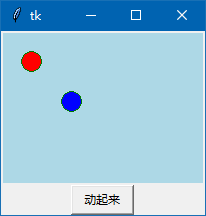```from tkinter import *
import time

def ok():  # 点击按钮，由yn变量决定是暂停还是继续动起来
global yn
if yn == True:
yn = False
but1['text'] = '  动起来  '
else:
yn = True
but1['text'] = '  暂 停  '
ballmove()  # 小球继续动起来

def ballmove():  # 小球在画布四周不停反弹
global x1, y1, x2, y2, yn

while yn:  # 由yn变量来控制小球是否移动

can1.move(xq1, x1, y1)         # 初始化,小球1,红球，向右下角移动
can1.move(xq2, x2, y2)         # 初始化,小球2，蓝球，向右上角移动

wz1 = can1.coords(xq1)   # 获取小球1的位置，一个4个元素的元组

if wz1 <= 0:          # 侦测球1是否超过画布左方
x1 = step
if wz1 <= 0:          # 侦测球1是否超过画布上方
y1 = step
if wz1 >= can1width:        # 侦测球1是否超过画布右方
x1 = -step
if wz1 >= can1height:       # 侦测球1是否超过画布下方
y1 = -step

wz2 = can1.coords(xq2)   # 获取小球2的位置，一个4个元素的元组

if wz2 <= 0:          # 侦测球是否超过画布左方
x2 = step2
if wz2 <= 0:          # 侦测球是否超过画布上方
y2 = step2
if wz2 >= can1width:        # 侦测球是否超过画布右方
x2 = -step2
if wz2 >= can1height:       # 侦测球是否超过画布下方
y2 = -step2

can1.update()       # 刷新画布
time.sleep(speed)   # 可以控制移动速度

# 相撞判断：
IDS=can1.find_overlapping(wz1, wz1, wz1, wz1) # 小球1的位置坐标参数
# find_overlapping(外接四方形左上角坐标，外接四方形右下角坐标)，返回的坐标范围内的画面对象元组。
if len(IDS) >= 2: # 在同一个四方框时有2个以上画布对象，即为相撞
print('相撞了')
yn = False
but1['text']='  动起来  '
break       # 退出循环，让小球暂停移动

root = Tk()

can1width = 200       # 定义画布宽度
can1height = 150      # 定义画布高度
step = 2        # 小球1移动的步长,2个像素
step2 = 3       # 小球2移动的步长,3个像素
x1, y1 = 2, 2         # 小球1移动的初始步长
x2, y2 = 2, -2      # 小球2移动的初始步长
speed = 0.03    # 设置移动速度
yn = False      # yn的值来控制球是否移动
r = 10            # 2个小球的半径
# xz=False # 相撞的状态

can1 = Canvas(root, background='lightblue',
width=can1width, height=can1height)  # 创建画布
can1.pack()

but1 = Button(root, text="  动起来  ", command=ok)  # 创建按钮
but1.pack()

xq1 = can1.create_oval(20, 20, 20+2*r, 20+2*r, fill='red',
outline='green')  # 绘制小球1,红球，半径为r个像素
xq2 = can1.create_oval(60, 60, 60+2*r, 60+2*r, fill='blue',
outline='green')  # 绘制小球2,蓝球，半径为r个像素

def xq1_move(event):  # 鼠标拖动小球
can1.coords(xq1, event.x-r, event.y-r, event.x+r, event.y+r)
# 将鼠标当前位置转为小球的外接正方形的左上角和右下角坐标（小球的半径为r）

def xq2_move(event):  # 鼠标拖动小球
can1.coords(xq2, event.x-r, event.y-r, event.x+r, event.y+r)
# 将鼠标当前位置转为小球的外接正方形的左上角和右下角坐标（小球的半径为r）

can1.tag_bind(xq1, "<B1-Motion>", xq1_move)  # 小球1,鼠标按住移动事件
can1.tag_bind(xq2, "<B1-Motion>", xq2_move)  # 小球2,鼠标按住移动事件

root.mainloop()```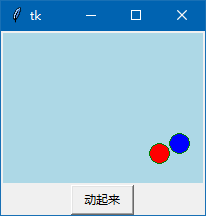```from tkinter import *
import time

def ok():  # 点击按钮，由yn变量决定是暂停还是继续动起来
global yn
if yn == True:
yn = False
but1['text'] = '  动起来  '
else:
yn = True
but1['text'] = '  暂 停  '
ballmove()  # 小球继续动起来

def ballmove():  # 小球在画布四周不停反弹
global x1, y1, x2, y2, yn

while yn:  # 由yn变量来控制小球是否移动

can1.move(xq1, x1, y1)         # 初始化,小球1,红球，向右下角移动
can1.move(xq2, x2, y2)         # 初始化,小球2，蓝球，向右上角移动

wz1 = can1.coords(xq1)   # 获取小球1的位置，一个4个元素的元组

if wz1 <= 0:          # 侦测球1是否超过画布左方
x1 = step
if wz1 <= 0:          # 侦测球1是否超过画布上方
y1 = step
if wz1 >= can1width:        # 侦测球1是否超过画布右方
x1 = -step
if wz1 >= can1height:       # 侦测球1是否超过画布下方
y1 = -step

wz2 = can1.coords(xq2)   # 获取小球2的位置，一个4个元素的元组

if wz2 <= 0:          # 侦测球是否超过画布左方
x2 = step2
if wz2 <= 0:          # 侦测球是否超过画布上方
y2 = step2
if wz2 >= can1width:        # 侦测球是否超过画布右方
x2 = -step2
if wz2 >= can1height:       # 侦测球是否超过画布下方
y2 = -step2

can1.update()       # 刷新画布
time.sleep(speed)   # 可以控制移动速度

# 相撞判断：
SS=pow( (((wz2+10)-(wz1+10))**2 + ((wz2+10)-(wz1+10))**2),1/2 ) # 小球每移动一次，即时求出2球的圆心之间的间距

print('ss=',SS) # 输出2球心之间的间距让大家看看

if SS<=20 :  # 20就是2个球的间距，等于或小于2球心之间的间距，就说明2球相撞了。
xz=True
print('相撞了')
yn = False
but1['text']='  动起来  '
break

root = Tk() # 源码来自wb98.com

can1width = 200       # 定义画布宽度
can1height = 150      # 定义画布高度
step = 2        # 小球1移动的步长,2个像素
step2 = 3       # 小球2移动的步长,3个像素
x1, y1 = 2, 2         # 小球1移动的初始步长
x2, y2 = 2, -2      # 小球2移动的初始步长
speed = 0.03    # 设置移动速度
yn = False      # yn的值来控制球是否移动
r = 10            # 2个小球的半径
# xz=False # 相撞的状态

can1 = Canvas(root, background='lightblue',
width=can1width, height=can1height)  # 创建画布
can1.pack()

but1 = Button(root, text="  动起来  ", command=ok)  # 创建按钮
but1.pack()

xq1 = can1.create_oval(20, 20, 20+2*r, 20+2*r, fill='red',
outline='green')  # 绘制小球1,红球，半径为r个像素
xq2 = can1.create_oval(60, 60, 60+2*r, 60+2*r, fill='blue',
outline='green')  # 绘制小球2,蓝球，半径为r个像素

def xq1_move(event):  # 鼠标拖动小球
can1.coords(xq1, event.x-r, event.y-r, event.x+r, event.y+r)
# 将鼠标当前位置转为小球的外接正方形的左上角和右下角坐标（小球的半径为r）

def xq2_move(event):  # 鼠标拖动小球
can1.coords(xq2, event.x-r, event.y-r, event.x+r, event.y+r)
# 将鼠标当前位置转为小球的外接正方形的左上角和右下角坐标（小球的半径为r）

can1.tag_bind(xq1, "<B1-Motion>", xq1_move)  # 小球1,鼠标按住移动事件
can1.tag_bind(xq2, "<B1-Motion>", xq2_move)  # 小球2,鼠标按住移动事件

root.mainloop()```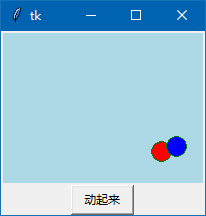```SS=pow( (((wz2+r)-(wz1+r))**2 + ((wz2+r)-(wz1+r))**2),1/2 )
# 小球每移动一次，即时求出2球的圆心之间的间距```

pow(A,1/2)  # 计算A2次方根

pow(A,1/3)  # 计算A3次方根

2球心之间的距离是一个数字问题，我稍讲解一下：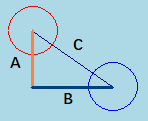B = 2的横坐标 - 1的横坐标 =  (wz2 + r) - (wz1 + r)

A = 2的纵坐标 - 1的纵坐标 =  (wz2 + r) - (wz1 + r)

C的平方=A的平方 + B的平方

C**2 = A**2 + B**2

2球心的距离为 C = 2次方根 [ A2次方 + B2次方）]

C= pow( (A**2 + B**2), 1/2 )

2球心之间的距离这个数字问题，我就讲解到这，不明白的，多看几遍吧。

```from tkinter import *
import time

def ok():  # 点击按钮，由yn变量决定是暂停还是继续动起来
global yn
if yn == True:
yn = False
but1['text'] = '  动起来  '
else:
yn = True
but1['text'] = '  暂 停  '
ballmove()  # 小球继续动起来

def ballmove():  # 小球在画布四周不停反弹
global x1, y1, x2, y2, yn, xz

while yn:  # 由yn变量来控制小球是否移动

can1.move(xq1, x1, y1)         # 初始化,小球1,红球，向右下角移动
can1.move(xq2, x2, y2)         # 初始化,小球2，蓝球，向右上角移动

wz1 = can1.coords(xq1)   # 获取小球1的位置，一个4个元素的元组

if wz1 <= 0:          # 侦测球1是否超过画布左方
x1 = step
if wz1 <= 0:          # 侦测球1是否超过画布上方
y1 = step
if wz1 >= can1width:        # 侦测球1是否超过画布右方
x1 = -step
if wz1 >= can1height:       # 侦测球1是否超过画布下方
y1 = -step

wz2 = can1.coords(xq2)   # 获取小球2的位置，一个4个元素的元组

if wz2 <= 0:          # 侦测球是否超过画布左方
x2 = step2
if wz2 <= 0:          # 侦测球是否超过画布上方
y2 = step2
if wz2 >= can1width:        # 侦测球是否超过画布右方
x2 = -step2
if wz2 >= can1height:       # 侦测球是否超过画布下方
y2 = -step2

can1.update()       # 刷新画布
time.sleep(speed)   # 可以控制移动速度

# 相撞判断：
# 小球每移动一次，即时求出2球的圆心之间的间距
SS = pow((((wz2+r)-(wz1+r))**2 +
((wz2+r)-(wz1+r))**2), 1/2)

print('ss=', SS)  # 输出2球心之间的间距让大家看看

if SS > 2*r:
xz = False  # 相距大于20个像素，肯定相撞状态为假

if SS <= 20 and xz == False:  # 20就是2个球的间距，等于或小于2球心之间的间距，就说明2球相撞了。
xz = True
print('相撞了')
yn = False
but1['text'] = '  动起来  '
break

root = Tk()　# 源码来自wb86.com

can1width = 200       # 定义画布宽度
can1height = 150      # 定义画布高度
step = 2        # 小球1移动的步长,2个像素
step2 = 3       # 小球2移动的步长,3个像素
x1, y1 = 2, 2         # 小球1移动的初始步长
x2, y2 = 2, -2      # 小球2移动的初始步长
speed = 0.03    # 设置移动速度
yn = False      # yn的值来控制球是否移动
r = 10            # 2个小球的半径
xz = False  # 相撞的状态

can1 = Canvas(root, background='lightblue',
width=can1width, height=can1height)  # 创建画布
can1.pack()

but1 = Button(root, text="  动起来  ", command=ok)  # 创建按钮
but1.pack()

xq1 = can1.create_oval(20, 20, 20+2*r, 20+2*r, fill='red',
outline='green')  # 绘制小球1,红球，半径为r个像素
xq2 = can1.create_oval(60, 60, 60+2*r, 60+2*r, fill='blue',
outline='green')  # 绘制小球2,蓝球，半径为r个像素

def xq1_move(event):  # 鼠标拖动小球
can1.coords(xq1, event.x-r, event.y-r, event.x+r, event.y+r)
# 将鼠标当前位置转为小球的外接正方形的左上角和右下角坐标（小球的半径为r）

def xq2_move(event):  # 鼠标拖动小球
can1.coords(xq2, event.x-r, event.y-r, event.x+r, event.y+r)
# 将鼠标当前位置转为小球的外接正方形的左上角和右下角坐标（小球的半径为r）

can1.tag_bind(xq1, "<B1-Motion>", xq1_move)  # 小球1,鼠标按住移动事件
can1.tag_bind(xq2, "<B1-Motion>", xq2_move)  # 小球2,鼠标按住移动事件

root.mainloop()```

Scavas组件要学的知识还有很多，本人也只是学了一点丁基础知识，以后学了很多的Scavas知识，再来讲解Scavas组件更深入的知识吧。

<< 上一篇 下一篇 >>